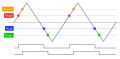#### hsilver

Joined Sep 6, 2016
6
My problem statement is to design a circuit to convert a triangular input signal of +/- 10 V amplitudes to a square wave output with +/- 13 V amplitudes. The circuit also needs the capability to control the phase of the output signal relative to the input signal in the range of 0 degrees to 90 degrees.

Im stuck on how to approach designing a circuit to allow for a variable phase shift of 0 degrees to 90 degrees. I am using a 10 K ohm potentiometer but dont know how I should pick my Vref or R1 (to get a Vs of 90 degrees) as seen in the picture attached.[/IMG]#### RBR1317

Joined Nov 13, 2010
712
Rather than jumping into designing the circuit, it might be helpful to first analyze the problem. Draw the triangular input on graph paper, then overlay the square wave output. From this you can get comparator switching points. Is a symmetric square wave output required? If Vref is adjustable, is a negative analog to Vref also necessary? Is a logic gate required for the output, or can open-collector comparator outputs be cleverly connected to provide the output function? Draw the comparator outputs on the graph. But draw the input & output waveforms first, then you'll have a better idea of what signal processing circuitry is needed.

#### RBR1317

Joined Nov 13, 2010
712
Here is my impression of the problem description. When using the term "Vref" it suggests using a comparator to establish the switching point with respect to Vref. However, a Schmitt Trigger is usually described in terms of threshold voltages which are established by the amount of positive feedback applied (usually determined by the relative value of the feedback resistor.) Since in this particular problem the switching points are symmetric around the zero voltage level, would your value of Vref be expected to equal zero?•hsilver

#### hsilver

Joined Sep 6, 2016
6
Here is my impression of the problem description. When using the term "Vref" it suggests using a comparator to establish the switching point with respect to Vref. However, a Schmitt Trigger is usually described in terms of threshold voltages which are established by the amount of positive feedback applied (usually determined by the relative value of the feedback resistor.) Since in this particular problem the switching points are symmetric around the zero voltage level, would your value of Vref be expected to equal zero?
View attachment 116337
To my understanding of the problem, your impression of the signal is correct.

It may not be necessary to have a Vref but my thought processes was to obtain an offset using a voltage divider and a potentiometer to get the variable phase shift. That is where I am getting confused. My professor breezed through this topic and I dont have much understanding on schmitt triggers. I believe I found the threshold voltages in my attempt.